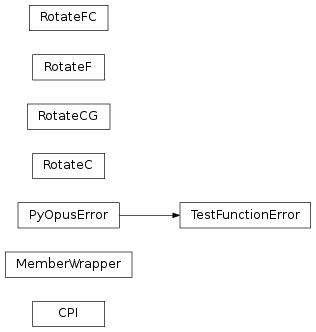# 5.1. `pyopus.problems.cpi` — Common problem interface¶Common problem interface (PyOPUS subsystem name: CPI)

Common problem interface (CPI) is a picklable dictionary containing all the information that is needed to describe an optimization problem.

The dictionary contains the following members:

• `setup` – Setup function. If not `None` this function must be called just before the problem is evaluated for the first time.
• `name` – problem name
• `n` – number of variables
• `m` – number of nonlinear constraints
• `x0` – the initial point
• `f` – returns the cost function value
• `g` – returns the cost function gradient
• `c` – returns the vector of nonlinear constraints
• `cg` – returns the Jacobian of the constraint functions, one row corresponds to one constraint, columns represent variables
• `fc` – returns the cost function value and the constraint function values
• `xlo` – a vector of lower bounds on variables
• `xhi` – a vector of upper bounds on variables
• `clo` – a vector of lower bounds on nonlinear constraints
• `chi` – a vector of upper bounds on nonlinear constraints
• `fmin` – best known minimum function value
• `xmin` – best known minimum
• `info` – additional problem information dictionary (suite dependent)

Lower and upper bounds are always defined. If some bound is not defined, it is set to `+Inf` or `-Inf`. If some memeber is not available it is set to `None`.

If the `setup` member is not `None` the problem belongs to a suite where multiple problems share internal variables. For such problems the setup function should be called just before the first problem evaluation. If later the setup function of a different problem from the same suite is called the values of the shared internal variables change and all subsequent evaluations of the previous problem result in incorrect values.

This class is inherited by optimization problems that support CPI. The `cpi()` method returns the CPI dictionary of the problem.

This module is independent of PyOPUS, meaning that it can be taken as is and used as a module in some other package.

Classes that inherit this class must reimplement the `cpi()` method.

class `pyopus.problems.cpi.``MemberWrapper`(obj, memberName)

Wraps a member function in a callable object.

obj is the object and memberName is the name of the member to wrap.

`pyopus.problems.cpi.``rotateProblem`(itf, Q, extremeBarrierBounds=False)

Rotate a problem described by itf using orthogonal matrix Q.

Feeds all functions with Qx instead of x.

Converts bounds to constraints.

Treats rotated bounds with extreme barrier approach if extremeBarrierBounds is `True`. If a bound is violated the returned function value is +Inf and the constraint values are an empty array ([]).

If extremeBarrierBounds is `False` the function and the constraints are evaluated even for points that violate bounds.

Example file cpi.py in folder demo/problems/

```# Demo of the Common Problem Interface

from pyopus.problems import mgh, glbc, lvns, mwbm
import numpy as np

if __name__ == '__main__':
# Build a list of problems from various collections
problems=[
# A More-Garbow-Hillstrom problem
mgh.ExtendedRosenbrock(n=10),
# Luksan-Vlcek problems
lvns.UNS("ElAttar"),
lvns.LCMM("PENTAGON"),
# A global optimization problem
glbc.Rastrigin(n=30),
# All variants of the More-Wild problem 9
mwbm.MWBM(9, problemType=0), # Smooth
mwbm.MWBM(9, problemType=1), # Piecewise-smooth
mwbm.MWBM(9, problemType=2, epsilon=1e-3), # Deterministically noisy
mwbm.MWBM(9, problemType=3, epsilon=1e-3), # Stochastically noisy
]

# Try adding some CUTEr problems
try:
from pyopus.problems.cuter import CUTEr

# Set these to True if you want to rebuild problems and do it quietly
rebuild=True
quiet=True

problems.extend([
CUTEr("HS71",  forceRebuild=rebuild, quiet=quiet),
CUTEr("HS80",  forceRebuild=rebuild, quiet=quiet),
CUTEr("ROSENBR",  forceRebuild=rebuild, quiet=quiet),
# Store as ARWHEAD_10 in cache, use N=10
CUTEr("ARWHEAD",  "ARWHEAD_10",  sifParams={ "N": 10 },   forceRebuild=rebuild, quiet=quiet),
])

except:
print("CUTEr not available")

for problem in problems:
# Get common problem interface
cpi=problem.cpi()

# Some problems require an initialization
# (call the 'setup' member of the cpi structure)
if cpi['setup'] is not None:
cpi['setup']()

# get dimension and number of constraints
n=cpi['n']
m=cpi['m']

# Get initial point
if cpi['x0'] is not None:
x0=cpi['x0']
else:
# Use the center of bounds on x (e.g. for glbc) as x0 when
# x0 is not available
x0=(cpi['xlo']+cpi['xhi'])/2

# Evaluate f at initial point
f0=cpi['f'](x0)

# Evaluate constraints
if m>0:
c0=cpi['c'](x0)

# Get bounds on constraint functions
cl=cpi['clo']
ch=cpi['chi']

# Cumulative constraint violation
h0=(np.where(c0<cl, cl-c0, 0.0)+np.where(c0>ch, c0-ch, 0.0)).sum()

# Print summary
print("%25s n=%2d: f0=%12.3e" % (cpi['name'], n, f0), end='')
if m>0:
print(" constrained, m=%2d, h0=%12.3e" % (m, h0))
else:
print()

```